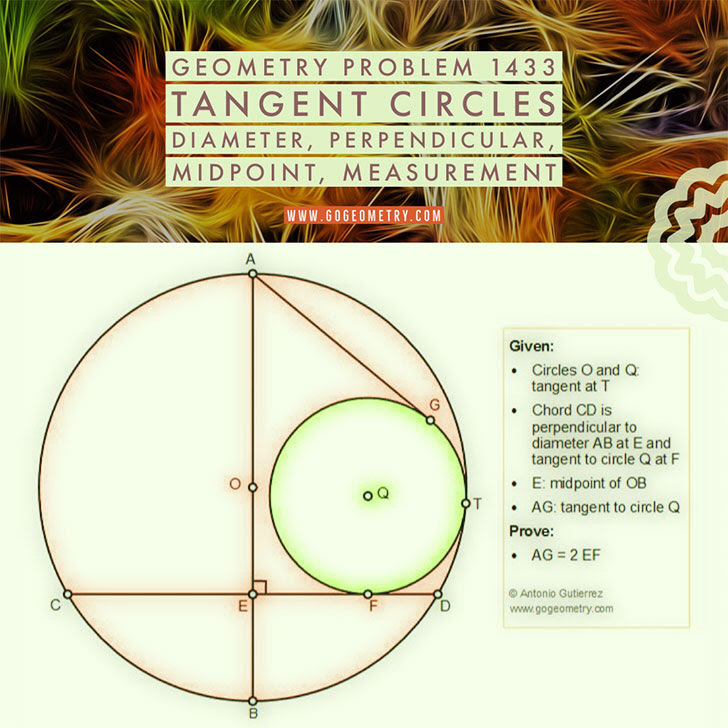## Sunday, May 12, 2019

### Geometry Problem 1433: Tangent Circles, Diameter, Perpendicular, Midpoint, Measurement, Poster

Geometry Problem. Post your solution in the comment box below.
Level: Mathematics Education, High School, Honors Geometry, College.

Details: Click on the figure below.1.https://photos.app.goo.gl/WkovAxHYaaVhSUNF8
connect QF , TB, TA
Let QF meet circle Q at H
Since circle O tangent to circle Q at T so O, Q,T are collinear
Triangles OBT and QFT are isosceles with ∠ (BOT)= ∠ (FQT)
So ∠ (OTB)= ∠ (QTF) => B, F, T are collinear
In the same way we also have A,H,T are collinear
Triangle BEF and BTA are similar ( case AA)
So AT=EF. (AB/BF)….(1)
Triangle BEF similar to HKA ( case AA)
So AH=BF .(KH/BE)=BF.(EF/BE)…(2)
Multiply (1) by (2) => AT. AH= AG^2= EF.(AB/BF). (BF/BE).EF
AG^2= (AB/BE). EF^2= 4 EF^2
So AG= 2 EF

2.OCBD being a rhombus, Tr. ACD is equilateral

However circle Q is not unique.

Hence Peter's proof above does not/ need not use this fact

3.the fact that E is the midpoint of OD ( or AB/BE=4) is implicitly indicate that ACD is equilateral.
In other word , if ACD is not equilateral , AG will not equal 2.EF

Peter Tran